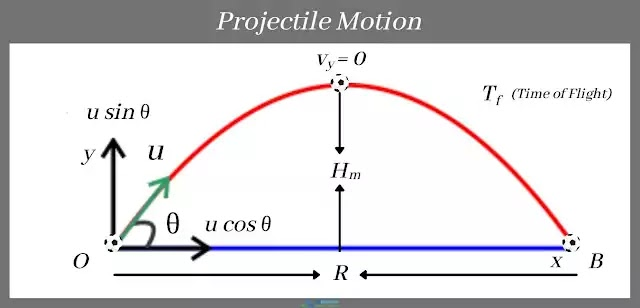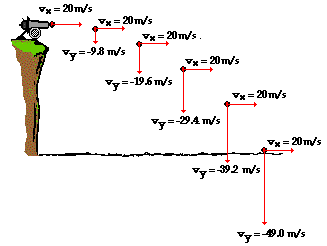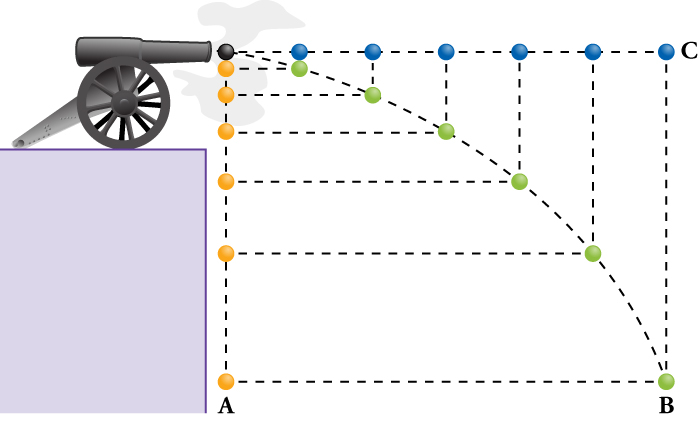# Projectile Motion Study Guide

INTRODUCTION

What is common between a bullet fired from a gun, a basketball shot from the end of a court, and a football kicked towards a goal? These objects are given an initial momentum to make it to a target. However, their path to the target is never straight. When shooting a basketball, you instinctively throw the ball higher while visualizing a curved path into the basket. Why the curve? Why can’t you send all these objects in a straight line to their targets? Let’s find out!Source

### WHAT IS A PROJECTILE?

Any object thrown or shot into the air and continues to fall only under the influence of gravitational acceleration is known as a projectile.

• The path is taken by a projectile from the moment it is shot is called a trajectory.
• While the primary force acting on a projectile is gravity, air creates some resistance to its motion called drag.

Air resistance can significantly change the trajectory of a projectile; however, it is ignored in most introductory physics problems.

### MOTION OF A PROJECTILE

It is important to divide its motion into vertical and horizontal components to analyze projectile motion. Consider a cannon shooting a ball at 20 m/s horizontally:Source

The moment the ball leaves the cannon, it begins to fall under the influence of gravity. The gravitational acceleration (g) increases the vertical component of velocity every second, as shown by the vector diagram in the figure. Under the absence of gravity, the ball would continue to fly horizontally in a straight line.

### WHAT CREATES THE CURVE IN THE TRAJECTORY?

When studying projectile motion in two dimensions, it is essential to understand that the vertical and horizontal components of motion of the object don’t influence one another. Consider the cannon shown in the above figure. Under the influence of gravity, if you were to shoot a cannonball horizontally and drop a cannonball simultaneously from the same position, both the balls would hit the ground at the same time.Source

In other words, the time of flight of projectile for both the balls is exactly the same! The orange ball has a vertical component of motion, but its horizontal component is zero. On the other hand, the green ball continuously travels further with a horizontal component of motion while still falling under the influence of gravity and traces a parabolic curve.

## CONCLUSION:

• When objects launched into the air fall only under the influence of gravity, they are called projectiles.
• The path traced by projectiles is called a trajectory.
• The vertical and horizontal components of a projective motion do not influence one another.

### FAQs:

1. What are the three types of projectile motion?

The three types of projectile motion examples include:

• Horizontal projectile motion
• Vertical projectile motion
• Oblique projectile motion (non horizontally launched projectiles)

2. What is projectile motion?

Projectile motion is the motion of an object solely under the influence of gravity. It includes the motion of all objects that are given an initial velocity and allowed to fall under gravity.

We hope you enjoyed studying this lesson and learned something cool about Projectile Motion! Join our Discord community to get any questions you may have answered and to engage with other students just like you! We promise, it makes studying much more fun! 😎

## SOURCES:

1. Projectile Motion. https://www.ck12.org/physics/projectile-motion/lesson/Projectile-Motion-MS-PS/?referrer=concept_details. Accessed 10 Feb 2022.
2. Projectile Motion. https://opentextbc.ca/openstaxcollegephysics/chapter/projectile-motion/. Accessed 10 Feb 2022.
3. 4.3 Projectile Motion. https://courses.lumenlearning.com/suny-osuniversityphysics/chapter/4-3-projectile-motion/. Accessed 10 Feb 2022.
4. Projectile Motion. https://byjus.com/physics/projectile-motion/#parabolic-motion-of-projectiles. Accessed 10 Feb 2022.
]]>